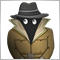# EA MT4 - Moving average on momentum12

Hi guys,

I'm trying to calculate a moving average over momentum indicator but EA crash at the boot of the back test because of the buffer I think ... someone has a better idea how to solve this ... I'm trying to calculate the moving average in the wrong way i think...

```//--- Get MOMENTUM and MA_Momentum
double   momentum_val = iMomentum(NULL,0,10,PRICE_WEIGHTED,0);
double   momentum_array[];

for(int i = 13; i >= 0; i--)
{
momentum_array[i] = iMomentum(NULL,0,10,PRICE_WEIGHTED,i);
}

double   ma_moment_val = iMAOnArray(momentum_array,0,14,0,MODE_SMA,0);```

tx a lot7859

`double    momentum_array;`

Or

```double    momentum_array[];
if( ArrayResize(momentum_array, 14) < 14 )
return;```12

Konstantin Nikitin:

Or

wow.... just it:

double    momentum_array;

tx a lot guy7859

vazsier:

wow.... just it:

double    momentum_array;

tx a lot guy

Yes, looked. But the meaning itself, think, is understandable